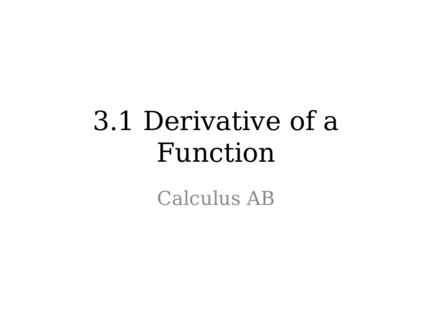# Derivative of a FunctionContributed by:Here we will be discussing the basics of a derivative of functions.
1. 3.1 Derivative of a
Function
Calculus AB
2. Vocab/Formulas
• Derivative:
– From 2.4 we defined slope of a curve of y = f(x) at
a point where x=a as
– When it exists, this limit is called the derivative
of f at a. f’(x) is the derivative of the function.
• Differentiable: a function is differentiable
at a point when the f’(x) exists
– If the function is differentiable at every point in
the domain, it is a differentiable function
3.
4.
5. Example 1: Differentiate
6.
7. Alternate Definition
• Derivative at a point x=a:
(a,
f(a))
a
8. Example 3: Use the alternative
derivative formula to differentiate at x = a
9. Example 4: Graph the derivative of
the given function.
10. Example 5: Sketch f given the
following information:
a.) f(0)=0 b.) The graph below is f’ c.) f is
continuous
11. Vocab
• Right-Hand Derivative:
• Left-Hand Derivative:
12. Example 6: Show that there are LH
& RH derivatives at x = 0 but no derivative
13. Pg. 105
1-18, 21